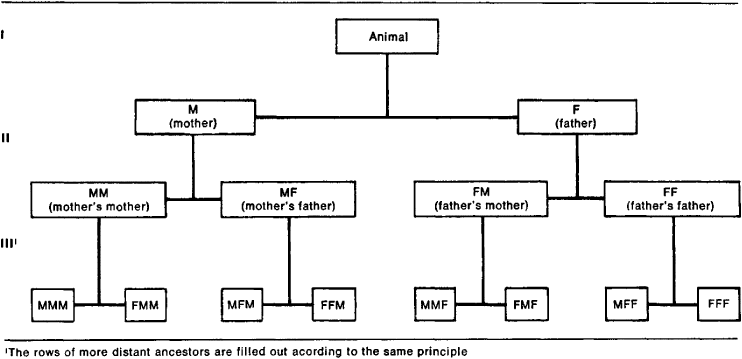# Genus of a Surface

The following article is from The Great Soviet Encyclopedia (1979). It might be outdated or ideologically biased.

## Genus of a Surface

a number characterizing the connectivity of a surface. Every closed orientable surface is topo-logically equivalent to a sphere with ρ handles. The number ρ is called the genus of such a surface. Thus, a sphere is a surface of genus 0, a torus has the genus 1, and a pretzel with two holes has the genus 2.

By means of 2p pairs of closed cuts, a surface of genus ρ can be transformed into a simply connected surface, that is, a surface divisible into two parts by an arbitrary closed curve other than one lying on the boundary of the surface. For example, a torus can be transformed into a simply connected surface by cuts along a meridian and a parallel. If the surface is a polyhedronFigure 1. Genealogical table

with k2 faces, k1 edges, and k0 vertexes, then its Euler characteristic is k2 – k1 + k0 = 2(1 – p).

A closed nonorientable surface is topologically equivalent to a sphere that has had h disks replaced by cross-caps; the number h is called the genus of such a surface.